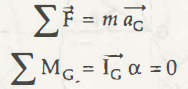The translation of a rigid body can be explained in rectilinear and curvilinear form. Every line of a translating body remains parallel. In case of a rectilinear body movement all points remain in the straight form. This straight line formation explains that there is no angle formed and thus the measurement of an angle will be zero.

If you take the translation of a body in a curvilinear form, then you will get the movement of the body on a curved path. Curved path means congruent path here. This is the prime reason that in translation motion there is no angle formation.

So, for the equationIt means the acceleration or angular acceleration alpha is 0. In both the conditions no angle is there. The value of its angular momentum is also zero.

KE for translation also depends on the proper knowledge of translation of a rigid body. So, in case of rigid body translation it is important to understand fundamentals in a proper way.

Links of Next Mechanical Engineering Topics:-### Customer Reviews

My Homework Help
Rated 5.0 out of 5 based on 510 customer reviews at